Download Presentation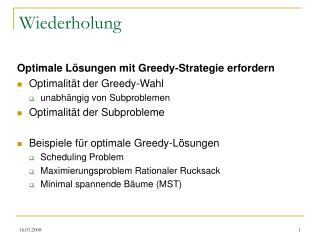Wiederholung

# Wiederholung - PowerPoint PPT Presentation

Wiederholung. Optimale Lösungen mit Greedy-Strategie erfordern Optimalität der Greedy-Wahl unabhängig von Subproblemen Optimalität der Subprobleme Beispiele für optimale Greedy-Lösungen Scheduling Problem Maximierungsproblem Rationaler Rucksack Minimal spannende Bäume (MST).I am the owner, or an agent authorized to act on behalf of the owner, of the copyrighted work described.
Download Presentation## Wiederholung

An Image/Link below is provided (as is) to download presentation

Download Policy: Content on the Website is provided to you AS IS for your information and personal use and may not be sold / licensed / shared on other websites without getting consent from its author.While downloading, if for some reason you are not able to download a presentation, the publisher may have deleted the file from their server.

- - - - - - - - - - - - - - - - - - - - - - - - - - E N D - - - - - - - - - - - - - - - - - - - - - - - - - -
Presentation TranscriptWiederholung

Optimale Lösungen mit Greedy-Strategie erfordern

• Optimalität der Greedy-Wahl
• unabhängig von Subproblemen
• Optimalität der Subprobleme
• Beispiele für optimale Greedy-Lösungen
• Scheduling Problem
• Maximierungsproblem Rationaler Rucksack
• Minimal spannende Bäume (MST)

TexPoint fonts used in EMF.

Read the TexPoint manual before you delete this box.: AAAA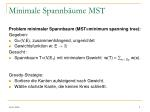Minimale Spannbäume MST

Problem minimaler Spannbaum (MST=minimum spanning tree):

Gegeben:

• G=(V,E), zusammenhängend, ungerichtet
• Gewichtsfunktion w: E !R

Gesucht :

• Spannbaum T=(V,ET) mit minimalem Gewicht w(T) = e 2 ET w(e).

Greedy-Strategie:

• Sortiere die Kanten aufsteigend nach Gewicht.
• Wähle nächste Kante, die keinen Kreis schließt.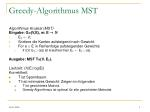Greedy-Algorithmus MST

Algorithmus Kruskal (MST)

Eingabe: G=(V,E), w: E ! N

• ETÃ;;
• Sortiere die Kanten aufsteigend nach Gewicht.
• For e 2 E in Reihenfolge aufsteigenden Gewichts
• If ((V, ET [ {e}) ist kreisfrei) then ETÃ ET[ {e}.

Ausgabe: MST T=(V, ET).

Laufzeit: O(|E| log|E|)

Korrektheit:

• T ist Spannbaum.
• T hat minimales Gewicht: Zeigen im folgenden
• T ist gewichtetes Matroid.
• Greedy-Algorithmus ist optimal für alle gewichteten Matroide.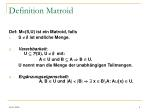Definition Matroid

Def: M=(S,U) ist ein Matroid, falls

• S ; ist endliche Menge.
• Vererbbarkeit: U µP(S), U ; mit:

A 2 U und B µ A ) B 2 U.

U nennt man die Menge der unabhängigen Teilmengen.

• Ergänzungseigenschaft: A, B 2 U und |A| < |B| )9 x 2 BnA: A[{x} 2 U.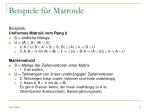Beispiele für Matroide

Beispiele:

Uniformes Matroid vom Rang k

• S = endliche Menge
• U = {A µ S | |A| · k}
• 2. A 2 U, B µ A ) B µ S, |B| · |A| · k ) B 2 U
• 3. A, B 2 U mit |A| < |B| · k )9 {x} 2 BnA ) |A [ {x}| · k ) A 2 U

Matrixmatroid

• S = Menge der Zeilenvektoren einer Matrix
• 1. S ist endlich.
• U = Teilmengen von linear unabhängigen Zeilenvektoren
• 2. Teilmengen linear unabh. Vektoren sind linear unabhängig.
• 3. Seien A, B 2 U mit |A| < |B|.

Es gibt in B einen Vektor, der linear unabhängig zu A ist.

(Steinitzscher Austauschsatz, Basisergänzungssatz)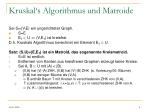Kruskal‘s Algorithmus und Matroide

Sei G=(V,E) ein ungerichteter Graph.

• S=E
• ET2 U , (V,ET) ist kreisfrei.

D.h. Kruskals Algorithmus berechnet ein Element ET2 U.

Satz: (S,U)=(E,ET) ist ein Matroid, das sogenannte Kreismatroid.

• S=E ist endlich.
• Entfernen von Kanten aus kreisfreien Graphen erzeugt keine Kreise.
• Sei A, B 2 U mit |A| < |B|.
• (V,A) hat |V|-|A| ZHK, (V,B) hat |V|-|B| ZHK (s. Vorlesung 06: Bäume)
• (V,B) hat weniger Bäume als (V,A).

) B enthält Baum T mit Knoten in verschiedenen ZHK von (V,A).

• T zusammenhängend: 9 x=(u,v) 2 T: u,v in verschiedenen ZHK von A.

) (V, A [ {x}) ist kreisfrei.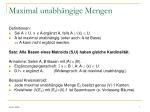Maximal unabhängige Mengen

Definitionen:

• Sei A 2 U. x  A ergänzt A, falls A [ {x} 2 U.
• A ist maximal unabhängig (oder auch: A ist Basis)

, A kann nicht ergänzt werden.

Satz: Alle Basen eines Matroids (S,U) haben gleiche Kardinalität.

Annahme: Seien A, B Basen mit |A| < |B|.

• Ergänzungseigenschaft: 9 x 2 B n A: A [ {x} 2 U.
• D.h. x ergänzt A. (Widerspruch: A ist maximal unabhängig.)

Beispiel Kreismatroid:

• Jede maximal unabhängige Menge ET besitzt genau |V|-1 Kanten.
• Kreisfreier (V,ET) mit |ET|=|V|-1 ist Spannbaum (s. Vorlesung Bäume).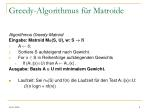Greedy-Algorithmus für Matroide

Algorithmus Greedy-Matroid

Eingabe: Matroid M=(S, U), w: S !N

• A Ã;;
• Sortiere S aufsteigend nach Gewicht.
• For x 2 S in Reihenfolge aufsteigenden Gewichts
• If (A[{x}2U) then A Ã A[{x} .

Ausgabe: Basis A 2 U mit minimalem Gewicht.

• Laufzeit: Sei n=|S| und f(n) die Laufzeit für den Test A[{x}2U:

O(n logn + nf(n))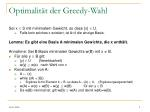Optimalität der Greedy-Wahl

Sei x 2 S mit minimalem Gewicht, so dass {x} 2 U.

• Falls kein solches x existiert, ist A=; die einzige Basis.

Lemma: Es gibt eine Basis A minimalen Gewichts, die x enthält.

Annahme: Sei B Basis minimalen Gewichts w(B) mit x  B.

• Für alle y 2 B gilt:
• {y} 2 U (Vererbbarkeit)
• w(y) ¸ w(x) nach Wahl von x
• Konstruktion von A:
• A Ã {x}
• while (|A| < |B|)
• Ergänze A mit Element b 2 B, so dass A [ {b} 2 U. (Ergänzungseigenschaft)
• w(A) = w(x) + w(B) – w(y) · w(B) für ein y 2 B
• Da w(B) minimal ist, gilt w(B) · w(A) und damit w(A)=w(B).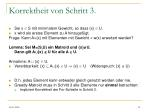Korrektheit von Schritt 3.
• Sei x 2 S mit minimalem Gewicht, so dass {x} 2 U.
• x wird als erstes Element zu A hinzugefügt.

Frage: Kann A={x} mit Elementen mit Gewicht < w(x) erweitert werden?

Lemma: Sei M=(S,U) ein Matroid und {x}U.

Dann gilt A[{x}  U für alle A 2 U.

Annahme: A [ {x} 2 U.

• Vererbbarkeit: {x} µ A[{x} 2 U ) {x} 2 U (Widerspruch: {x}  U)
• D.h. jedes Element kann entweder gleich genutzt werden oder nie.
• Greedy-Matroid muss in Schritt 3 alle Elemente nur einmal betrachten:
• Impliziert Korrektheit der For-Schleife in Schritt 3.Optimalität der Subprobleme

Lemma: Sei x das erste von Greedy-Matroid ausgewählte Element. Dann muss eine minimale Basis des Matroids M‘=(S‘,U‘) gefunden werden, wobei

• S‘ = {y 2 S | {x,y} 2 U}
• U‘ = {A µ Sn{x} | A[{x} 2 U}.
• A ist Basis von M mit x 2 A , A‘=A\{x} ist Basis von M‘
• Es gilt w(A) = w(A‘) + w(x).
• D.h. jede minimale Basis A für M liefert

eine minimale Basis A‘ für M‘ und umgekehrt.Zusammenfassen der Lemmata

Satz: Greedy-Matroid berechnet bei Eingabe M=(S,U) eine minimale Basis von M.

• Jedes Element y mit {y}  U braucht nicht betrachtet werden.
• Sei w(x) minimal mit {x} 2 U.
• Falls kein solches x existiert, ist A=; die einzige Basis.
• Greedy-Wahl: Es gibt eine optimale Lösung A mit x 2 A.
• Subproblem: Finde optimale Lösung im Matroid M‘.

Korollar: Alg. Kruskal berechnet einen minimalen Spannbaum.Minimierung versus Maximierung

Maximierungsproblem

• Gegeben: gewichtetes Matroid M=(S,U), w: S !N
• Gesucht: Basis von A mit maximalem Gewicht

1. Möglichkeit: Modifiziere Greedy-Matroid

• Sortiere absteigend
• Wähle greedy maximales Element, das A ergänzt.

2. Möglichkeit: Modizifiere Gewichtsfunktion w

• Übungsaufgabe

Warnung: Es gibt zahlreiche Probleme

• die optimal mit Greedy gelöst werden können, aber
• von denen keine Matroiddarstellung bekannt ist.